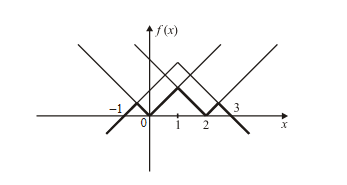# Examples On Differentiability Set-3

Go back to  'LCD'

Example - 23

A function  $$f:\mathbb{R} \to \mathbb{R}$$ satisfies $$\left| {f\left( x \right) - f\left( y \right)} \right| \le {\left| {x - y} \right|^3}$$   for all $$x,y \in \mathbb{R}.$$  Prove that $$f\left( x \right)$$  is a constant function.

Solution:    For a constant function f(x) = k, the tangent drawn at any point would be the same line y = k itself. Hence, the slope of tangent at any point (the derivative at any point) has the value 0.

Therefore, our aim in this question should be to somehow show that f(x) has derivative 0 at all points.

Let us evaluate the RHD at any x:

\begin{align}{\rm{RHD}}\,{\rm{at}}\,x = \mathop {\lim }\limits_{h \to 0} \frac{{f\left( {x + h} \right) - f\left( x \right)}}{h}\end{align}

Therefore,

\begin{align}\left| {{\rm{RHD}}\,{\rm{at}}\,x} \right|&= \left| {\mathop {\lim }\limits_{h \to 0} \frac{{f\left( {x + h} \right) - f\left( x \right)}}{h}} \right|\\&= \mathop {\lim }\limits_{h \to 0} \frac{{\left| {f\left( {x + h} \right) - f\left( x \right)} \right|}}{{\left| h \right|}}\\& \le \mathop {\lim }\limits_{h \to 0} \frac{{{{\left| {\left( {x + h} \right) - \left( x \right)} \right|}^3}}}{{\left| h \right|}} \,\,\,\,\,\,\,\,\,\,\,\,\left\{ {{\rm{Using}}\,{\rm{the}}\,{\rm{given}}\,{\rm{relation}}} \right\}\\& = \mathop {\lim }\limits_{h \to 0} {\left| h \right|^2} = 0\\ &\Rightarrow \left| {RHD} \right| \le 0 \end{align}

Since $$\left| {RHD} \right|$$   cannot be negative, it has to be 0.

$$\Rightarrow RHD = 0$$   for all x

Similarly, LHD = 0 for all x

$$\Rightarrow$$   f (x) is a constant function.

Example – 24

Let  f\left( x \right) = \left\{ {\begin{align}{}{x{e^{ - \left\{ {\frac{1}{{\left| x \right|}} + \frac{1}{x}} \right\}}},} {\;\;\;\;\; x \ne 0}\\{0\,\,\,\,\,\,\,\,\,\,\,\,\,\,\,\,,} {\;\;\;\;\;x = 0} \end{align}} \right\}  ,  Evaluate the continuity and differentiability of f (x)

Solution:    We should first of all write f (x) separately for x > 0  and x < 0;  using \left| x \right| = \left\{ {\begin{align}{}{x\,\,\,\,,} {\;\;\;\;\;x > 0}\\{ - x\,,} {\;\;\;\;\;x < 0}\end{align}} \right\}

f\left( x \right) = \left\{ {\begin{align}{} {x{e^{ - \frac{2}{x}}}} {\;\;\;\;\;x > 0}\\0 {\;\;\;\;\;x = 0}\\ x {\;\;\;\;\;x < 0}\end{align}} \right\}

At this juncture, we do not have sufficient knowledge to accurately plot the graph for x > 0;  we will hence follow the analytical approach.

The critical point is only x = 0

\begin{align}&{\rm{LHL}}\left( {{\rm{at}}\,x = 0} \right) = \mathop {\lim }\limits_{x \to {0^ - }} \left( x \right) = 0\\&{\rm{RHL}}\left( {{\rm{at}}\,x = 0} \right) = \mathop {\lim }\limits_{x \to {0^ + }} \left( {x{e^{ - 2/x}}} \right) = 0\,\,\,\,\,\left( {{\rm{Verify}}} \right)\\&{\rm{LHD}}\left( {{\rm{at}}\,x = 0} \right) = \mathop {\lim }\limits_{h \to 0} \frac{{f\left( { - h} \right) - f\left( 0 \right)}}{{ - h}}\\&\qquad\qquad\qquad\;\;{\rm{ }} = {\rm{ }}1\\&{\rm{RHD}}\left( {{\rm{at }}x\, = {\rm{0}}} \right) = \mathop {\lim }\limits_{h \to 0} \frac{{f\left( h \right) - f\left( 0 \right)}}{h}\\&\qquad\qquad\qquad\;\;{\rm{ }} = \mathop {\lim }\limits_{h \to 0} \frac{{h{e^{ - 2/h}}}}{h}\\&\qquad\qquad\qquad\;\;{\rm{ }} = {\rm{ }}0\end{align}

Therefore, this function is continuous at x = 0 (and everywhere else) but not differentiable at x = 0

Example - 25

If  $$f\left( x \right) = \min \left\{ {\left| x \right|,\left| {x - 2} \right|,2 - \left| {x - 1} \right|} \right\},$$ draw the graph of $$f\left( x \right)$$  and discuss its continuity and differentiability.

Solution:    To drawn the graph of f(x) , we plot the three ‘mod’ functuions on the same axis, and then take the minimum:It is immediately obvious that f(x) is continuous everywhere but non-differentiable at

\begin{align}x = - \frac{1}{2},\;0,\;1,\;2,\;\frac{5}{2}.\end{align}

## TRY YOURSELF – IV

Q. 1 If  f\left( x \right) = \left\{ {\begin{align}{}{\frac{{x\left( {3{e^{1/3}} + 4} \right)}}{{2 - {e^{}}}},}{\;\;\;\;\;x \ne 0}\\{\;0,\;}{\;\;\;\;\;x = 0}\end{align}} \right\}  find whether $$f\left( x \right)$$  is continuous and differentiable   at x = 0.

Q. 2 Test the differentiability of   f\left( x \right) = \left\{ {\begin{align}{}{\left| {x - 1} \right|\left( {[x] - x} \right),}{\;\;\;\;\;x \ne 1}\\{0,}{\;\;\;\;\;x = 0}\end{align}} \right\}\;{\rm{at}}\;x = 1

Q. 3 Given   $$\left| {f\left( x \right) - f\left( y \right)} \right| \le {\left| {x - y} \right|^2}\;{\rm{and}}\;f\left( 0 \right) = 1,\;{\rm{find}}\;f\left( x \right)$$

Q. 4 Let f\left( x \right) = \left\{ {\begin{align}{}{k\left\{ {1 - \frac{{\;\;\;\;\;\left| x \right|}}{l}} \right\},}{\left| x \right| \le l}\\{0\;\;\;\;\;\;,}{\;\;\;\;\;\left| x \right| > l}\end{align}} \right\} . Find the points where $$f\left( x \right)$$  is non-differentiable.

Q. 5  Let f\left( x \right) = \left\{ {\begin{align}{}{{x^k},}{\;\;\;\;\;x > 0}\\{0,}{\;\;\;\;\;x = 0}\\{ - {x^k},}{\;\;\;\;\;x < 0}\end{align}} \right\}    where k > 0. What are the possible values of k for which f(x) is differentiable at x = 0 ?